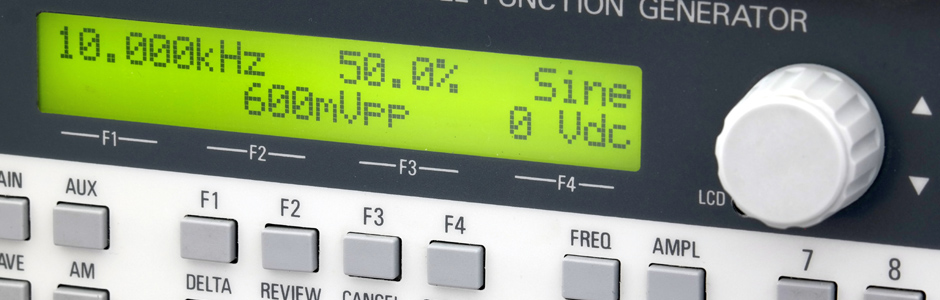•# Audio converter

### Convert voltage U (volts) to voltage level Lu (dBu)

Reference voltage U₀ = 0.7746 Volt ≡ 0 dBu
Voltage Urms Volt Voltage level Lrms dBu

### Convert voltage U (volts) to voltage level LV (dBV)

Reference voltage U₀ = 1 Volt ≡ 0 dBV
Voltage Urms Volt Voltage level Lu dBV

### Convert magnetic flux Φ0 (nWb/m) to voltage level Lu (dBu)

a) with a reference magnetic flux Φ0 = 514 nWb/m (0 dBu ≡ 0.7746 volts rms).
Magnetic flux Φ0 (nWb/m) Voltage level Lu dBu
b) with a reference magnetic flux Φ0 = 320 nWb/m (0 dBu ≡ 0.7746 volts rms).
Magnetic flux Φ0 (nWb/m) Voltage level Lu dBu
c) with a reference magnetic flux Φ0 = 257 nWb/m (0 dBu ≡ 0.7746 volts rms).
Magnetic flux Φ0 (nWb/m) Voltage level Lu dBu
d) with a reference magnetic flux Φ0 = 250 nWb/m (0 dBu ≡ 0.7746 volts rms).
Magnetic flux Φ0 (nWb/m) Voltage level Lu dBu
e) with a reference magnetic flux Φ0 = 200 nWb/m (0 dBu ≡ 0.7746 volts rms).
Magnetic flux Φ0 (nWb/m) Voltage level Lu dBu
f) with a reference magnetic flux Φ0 = 185 nWb/m (0 dBu ≡ 0.7746 volts rms).
Magnetic flux Φ0 (nWb/m) Voltage level Lu dBu
g) with a reference magnetic flux Φ0 = 160 nWb/m (0 dBu ≡ 0.7746 volts rms).
Magnetic flux Φ0 (nWb/m) Voltage level Lu dBu